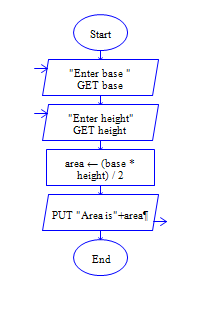# Java Program to find area of triangle

Get base width and height of triangle and find area of triangle

Sample Input 1:

4 5

Sample Output 1:

10

#### Flow Chart DesignStrongly recommended to Solve it on your own, Don't directly go to the solution given below.

public class Hello { public static void main(String args[]) { //Write your code here } }

#### Program or Solution

``` import java.util.*; class Triangle { public static void main(String args[]) { double base,height; double area; Scanner sc=new Scanner(System.in); System.out.println("Get base and height of Triangle and find area of Triangle"); System.out.println("Enter The Base:"); base=sc.nextInt(); System.out.println("Enter The Height:"); height=sc.nextInt(); area=0.5*base*height; System.out.println("The Output 1 Is:\n"+area); } } ```

#### Program Explanation

Get height and base of a triangle (using scanner class) Calculate area by multiplying product of height and base by 0.5 (area = base * height*0.5) print area (using system.out.println)